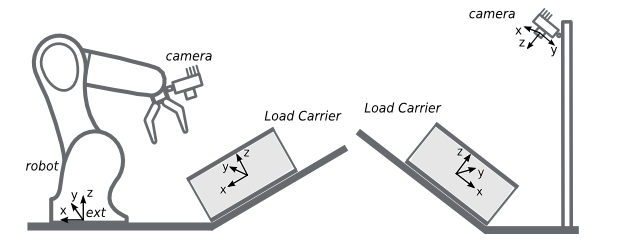# FAQs and troubleshooting¶

The load carrier rim is not visible in the depth image

One common case for missing the rim in depth images takes place when one of the edges of the load carrier is parallel to the baseline of the stereo system. It can be easily determined if this the case by slightly rotating the load carrier and observing if the orientation has an effect on the appearance of the object in the depth image.

The load carrier is not detected or not detected robustly

• Is the load carrier fully visible in both the left and right camera image?

• Does the load carrier rim appear in the depth image? (cfr. Setting up the scene)

• Is the load carrier inside the region of interest (if specified)?

• Are the dimensions of the load carrier model correct? (cfr. Configuring the load carrier)

The manufacturer’s dimensions might not be completely accurate. If the load carrier is not detected, we recommended to double check the configured dimensions. Additionally, one can try increasing the load_carrier_model_tolerance parameter (e.g. to the maximum value).

• The load carrier is not on a horizontal surface

• The load carrier is deformed

The load carrier is not placed on a horizontal surface

By default, ItemPick assumes that the load carrier is located on a horizontal surface. If that’s not the case, one needs to provide the load carrier orientation as a prior with the load carrier model.

This is currently not possible in the Web GUI’s ItemPick panel. Instead, it can be set via the REST-API interface.

Two sample cases are shown in Fig. 24 for the external pose frame (left) and the camera pose frame (right).Fig. 24 Load carrier not placed on a horizontal surface

In the left case of Fig. 24, the load carrier (tilted-load-carrier-ext) is rotated by an angle $$\theta$$ around the $$y$$ axis of the external coordinate system. The load carrier orientation is given by the following quaternion: $$\left[0, \sin(\theta/2), 0, \cos(\theta/2)\right]$$.

Request to the REST-API for configuring tilted-load-carrier-ext

Here we make the assumption that tilted-load-carrier-ext has the same dimensions of my-load-carrier-1 (cfr. Configuring the load carrier) and that the angle $$\theta$$ is 30 deg.

To trigger the set_load_carrier service via the REST-API for tilted-load-carrier-ext, one needs to send a PUT request to the URL http://<rc-visard-ip>/api/v1/nodes/rc_itempick/services/set_load_carrier, where <rc-visard-ip> should be replaced by the actual IP of the rc_visard.

The PUT body should include the following data, in JSON:

{
"args": {
"outer_dimensions": {
"x": 0.4,
"y": 0.3,
"z": 0.22
},
"inner_dimensions": {
"x": 0.37,
"y": 0.27,
"z": 0.215
},
"pose_frame": "external",
"pose": {
"orientation": {
"x": 0,
"y": 0.25882,
"z": 0,
"w": 0.96593
}
}
}
}
}


In the right case of Fig. 24, the load carrier (tilted-load-carrier-cam) is parallel to the image plane. Its orientation in the camera coordinate system is $$\left[\sqrt(2)/2, -\sqrt(2)/2, 0, 0\right]$$.

Request to the REST-API for configuring tilted-load-carrier-cam

Here we make the assumption that tilted-load-carrier-cam has the same dimensions of my-load-carrier-1 (cfr. Configuring the load carrier).

To trigger the set_load_carrier service via the REST-API for tilted-load-carrier-ext, one needs to send a PUT request to the URL http://<rc-visard-ip>/api/v1/nodes/rc_itempick/services/set_load_carrier, where <rc-visard-ip> should be replaced by the actual IP of the rc_visard.

The PUT body should include the following data, in JSON:

{
"args": {
"outer_dimensions": {
"x": 0.4,
"y": 0.3,
"z": 0.22
},
"inner_dimensions": {
"x": 0.37,
"y": 0.27,
"z": 0.215
},
"pose_frame": "camera",
"pose": {
"orientation": {
"x": 0.70711,
"y": -0.70711,
"z": 0,
"w": 0
}
}
}
}
}


One can try increasing the load_carrier_model_tolerance parameter (e.g. to the maximum value).

For significantly deformed load carriers, the detection algorithm might not provide reliable results. This can for example be the case of cardboard boxes after several uses. An alternative for such cases is to fix the load carrier placement and manually select a region of interest inside the load carrier.

This means that either the load carrier $$z$$ inner dimension is too large or the reconstruction of the load carrier floor is noisy.

To improve the detection result as shown in Fig. 25, two options are available:

• Decrease the load carrier $$z$$ inner dimension
• Increase the load_carrier_crop_distace parameter (recommended for noisy data)

Objects on the load carrier floor are not detected as load carrier content

This means that either the load carrier $$z$$ inner dimension is too small or the the load_carrier_crop_distace parameter is too large.

There are multiple load carriers of the same type in the scene

In the current implementation, ItemPick detects one load carrier with each compute_grasps or detect_load_carriers request.

If there are multiple load carriers of the same type in the scene, we recommended to specify one ore more regions of interest, each one including one load carrier instance.

My load carrier doesn’t move. How do I speed up my application?

In case of static load carriers, the processing time for getting grasp points inside the load carrier can be reduced, by avoiding detecting the same load carrier over multiple frames. The load carrier detection can be triggered once and then, for the next iterations, a region of interest inside the load carrier can be used instead.

There are no grasps detected on the objects

• Do objects appear in the depth image? (an additional pattern projector might be needed)
• Do the workpieces appear in the depth image without holes? (cfr. Configuring image parameters)
• Are the workpieces inside the region of interest (if specified)?
• Is the load carrier detected (if specified)?
• Is the object smaller than the cluster_maximum_dimension value?

There are too many grasps on one single object

The clustering_surface_max_rmse and cluster_maximum_curvature parameters should be increased.